# Form Operators and Functions#

Expressions in calculations, constraints, and relevants can contain operators and functions.

## Operators#

### Math operators#

Explanation

Example

+

\${salary_income} + \${self_employed_income}

-

subtraction

\${income} - \${expenses}

*

multiplication

\${bill} * 1.18

div

division

\${percent_int} div 100

mod

modulo (division remainder)

(\${even_number} mod 2) = 0

Warning

Math operators only work with numbers.

### Comparison operators#

Comparison operators are used to compare values. The result of a comparison is always `True` or `False`.

Explanation

Example

Notes

=

equal to

\${enrolled} = 'yes'

Can compare numbers or strings.

!=

not equal to

\${enrolled} != 'yes'

Can compare numbers or strings.

>

greater than

\${age} > 17

>=

greater than or equal to

\${age} >= 18

<

less than

\${age} < 65

<=

less than or equal to

\${age} <= 64

Warning

• The relational operators (`>`, `>=`, `<`, `<=`) only work with numbers.

### Boolean operators#

Boolean operators combine two `True` or `False` values into a single `True` or `False` value.

Explanation

Example

and

`True` if the expressions before and after are `True`

\${age} > -1 and \${age} < 120

or

`True` if either of the expressions before or after are `True`

\${age} < 19 or \${age} > 64

### Path operators#

Explanation

Example

Notes

.

current question's value

. >= 18

Used in constraints.

..

current question's parent group

position(..)

Used with `position()` to get a parent repeat instance's index.

Note

Formally, these are not operators but rather XPath references to the current node (`.`) and the parent node (`..`). XPath paths can be used to reference nodes of a form.

## Functions#

### Control flow#

if(expression, then, else)#

Returns `then` if `expression` evaluates to `True`. Otherwise, returns `else`.

position(xpath)#

Returns an integer equal to the 1-indexed position of the current node within the node defined by `xpath`.

Most often this is used in the form `position(..)` to identify the current iteration index within a repeat group.

XLSForm

type

name

label

repeat_count

calculation

note

person_list_note

begin_repeat

person

Member of household

text

name

Name

end_repeat

begin_repeat

person_details

Details

count(\${person})

calculate

current_name

indexed-repeat(\${name}, \${person}, position(..))

date

member_bday

Birthday of \${current_name}

end_repeat

once(expression)#

Returns the value `expression` if the question's value is empty. Otherwise, returns the current value of the question.

This can be used to ensure that a random number is only generated once, or to store the first value entered for a question in a way that is retrievable even if the response is changed later.

Warning

### Accessing response values#

Note

The response from most question types can be accessed using variables. Functions are needed for accessing responses to multi select questions and questions inside repeat groups.

#### Select questions#

selected(space_delimited_array, string)#

Returns `True` if `string` is a member of `space_delimited_array`, otherwise returns `False`.

Commonly used to determined if a specific choice was selected in a select question. (This is possible because a reference to a select question returns a space-delimited array of choice names.)

XLSForm

survey#

type

name

label

hint

relevant

constraint

select_multiple medical_issues

what_issues

Have you experienced any of the following?

Select all that apply.

select_multiple cancer_types

what_cancer

What type of cancer have you experienced?

Select all that apply.

selected(\${what_issues}, 'cancer')

select_multiple diabetes_types

what_diabetes

What type of diabetes do you have?

Select all that apply.

selected(\${what_issues}, 'diabetes')

begin_group

blood_pressure

selected(\${what_issues}, 'hypertension')

integer

systolic_bp

Systolic

. > 40 and . < 400

integer

diastolic_bp

Diastolic

. >= 20 and . <= 200

end_group

text

other_health

List other issues.

selected(\${what_issues}, 'other')

note

after_health_note

This note is after all health questions.

choices#

list_name

name

label

medical_issues

cancer

Cancer

medical_issues

diabetes

Diabetes

medical_issues

hypertension

Hypertension

medical_issues

other

Other

cancer_types

lung

Lung cancer

cancer_types

skin

Skin cancer

cancer_types

prostate

Prostate cancer

cancer_types

breast

Breast cancer

cancer_types

other

Other

diabetes_types

type_1

Type 1 (Insulin dependent)

diabetes_types

type_2

Type 2 (Insulin resistant)

selected-at(space_delimited_array, n)

Returns the string at the `n`th position of the `space_delimited_array`. (The array is zero-indexed.) Returns an empty string if the index does not exist.

This can be used to get the `name` of a selected choice from a multi-select question. (This is possible because a reference to a select question returns a space-delimited array of choice names.)

Note

If used to get a choice name from a select question, this function returns the `name`, not the `label`, of the selected choice. To get the label in the current language, use `jr:choice-name()`.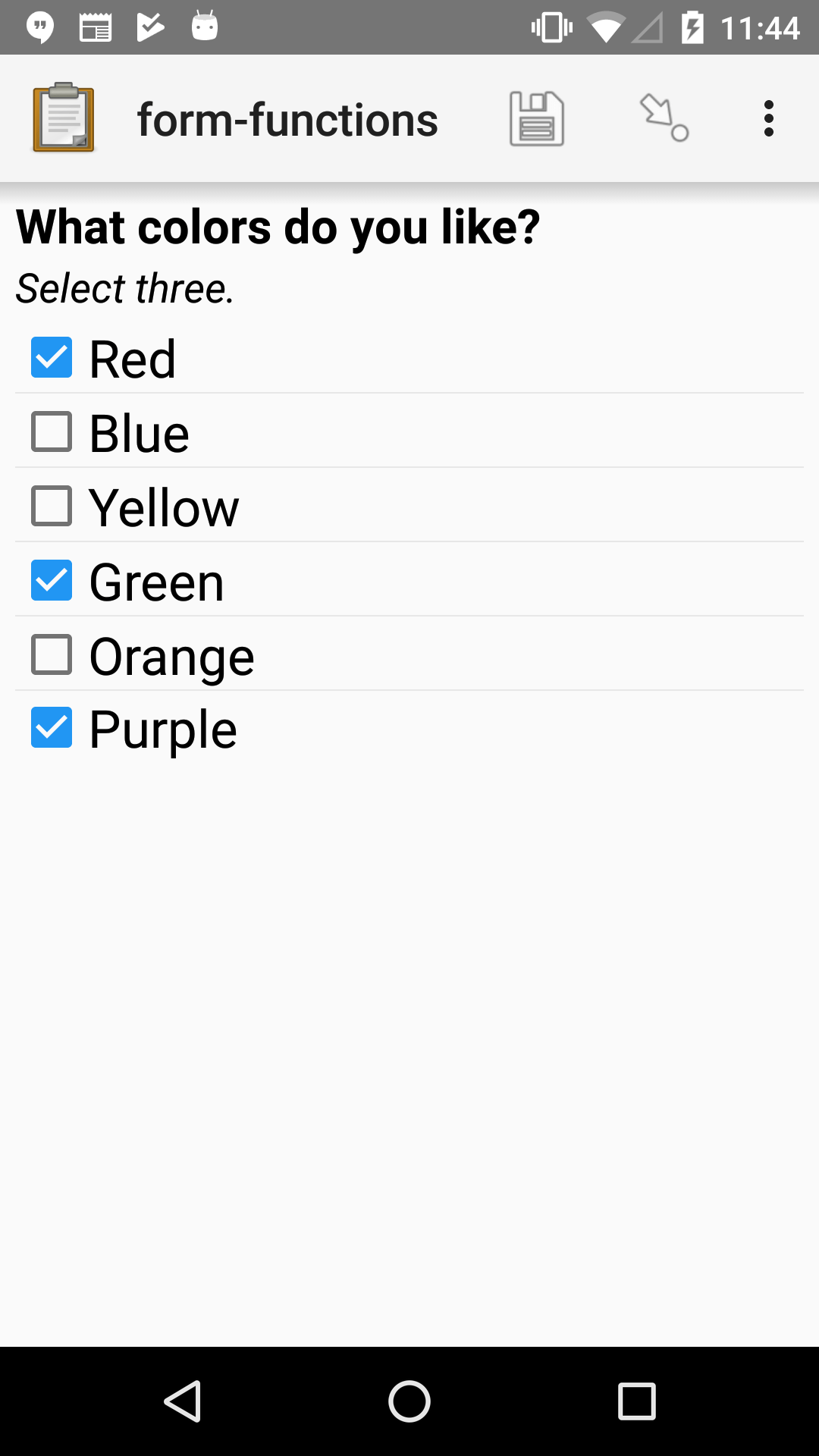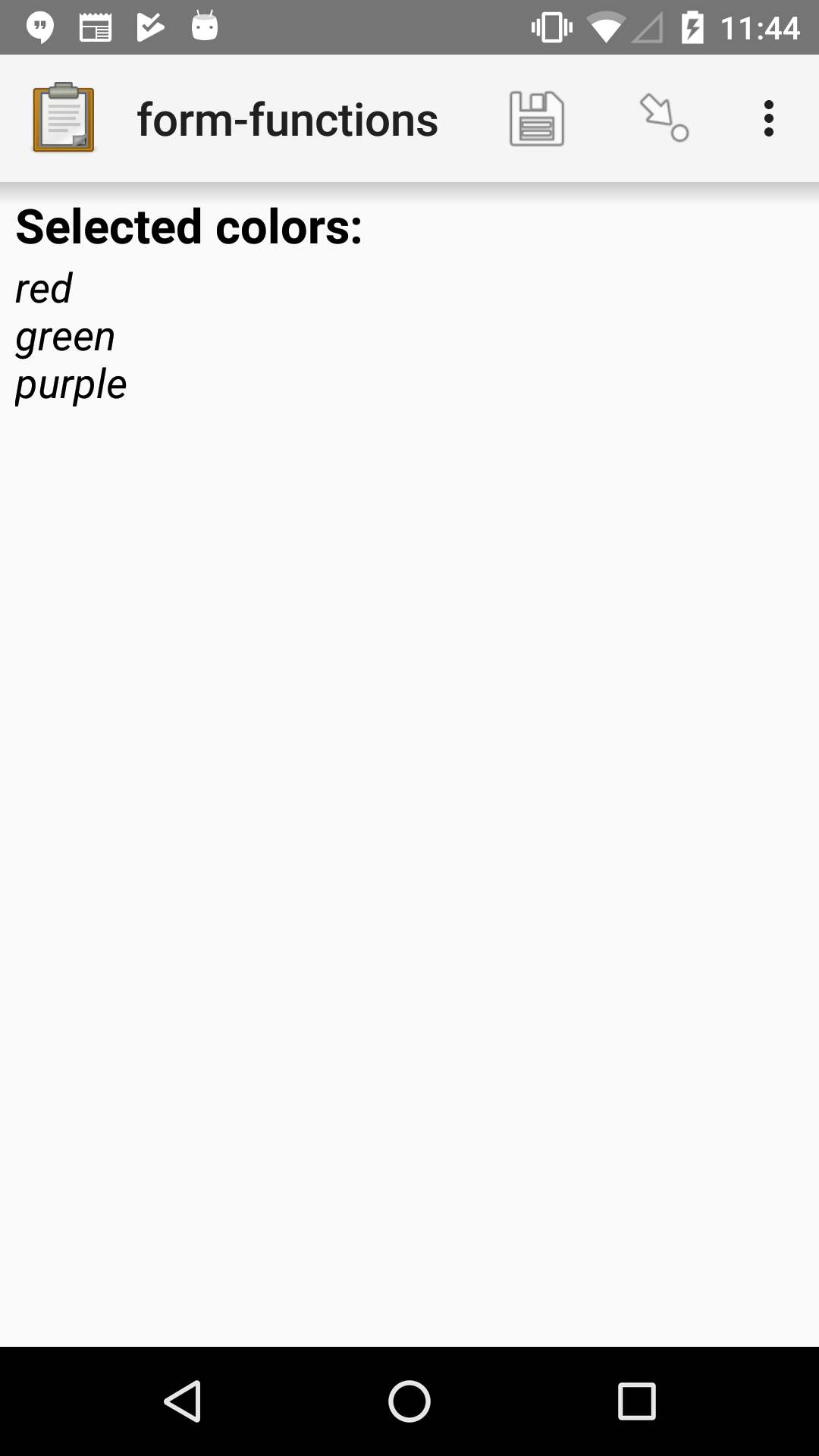XLSForm

survey#

type

name

label

hint

calculation

select_multiple colors

color_prefs

What colors do you like?

Select three.

calculate

color_0

selected-at(\${color_prefs}, 0)

calculate

color_1

selected-at(\${color_prefs}, 1)

calculate

color_2

selected-at(\${color_prefs}, 2)

note

color_note

Selected colors:

\${color_0} <br> \${color_1} <br> \${color_2}

choices#

list_name

name

label

colors

red

Red

colors

blue

Blue

colors

yellow

Yellow

colors

green

Green

colors

orange

Orange

colors

purple

Purple

count-selected(multi_select_question)

Returns the number of choices selected in `multi_select_question`.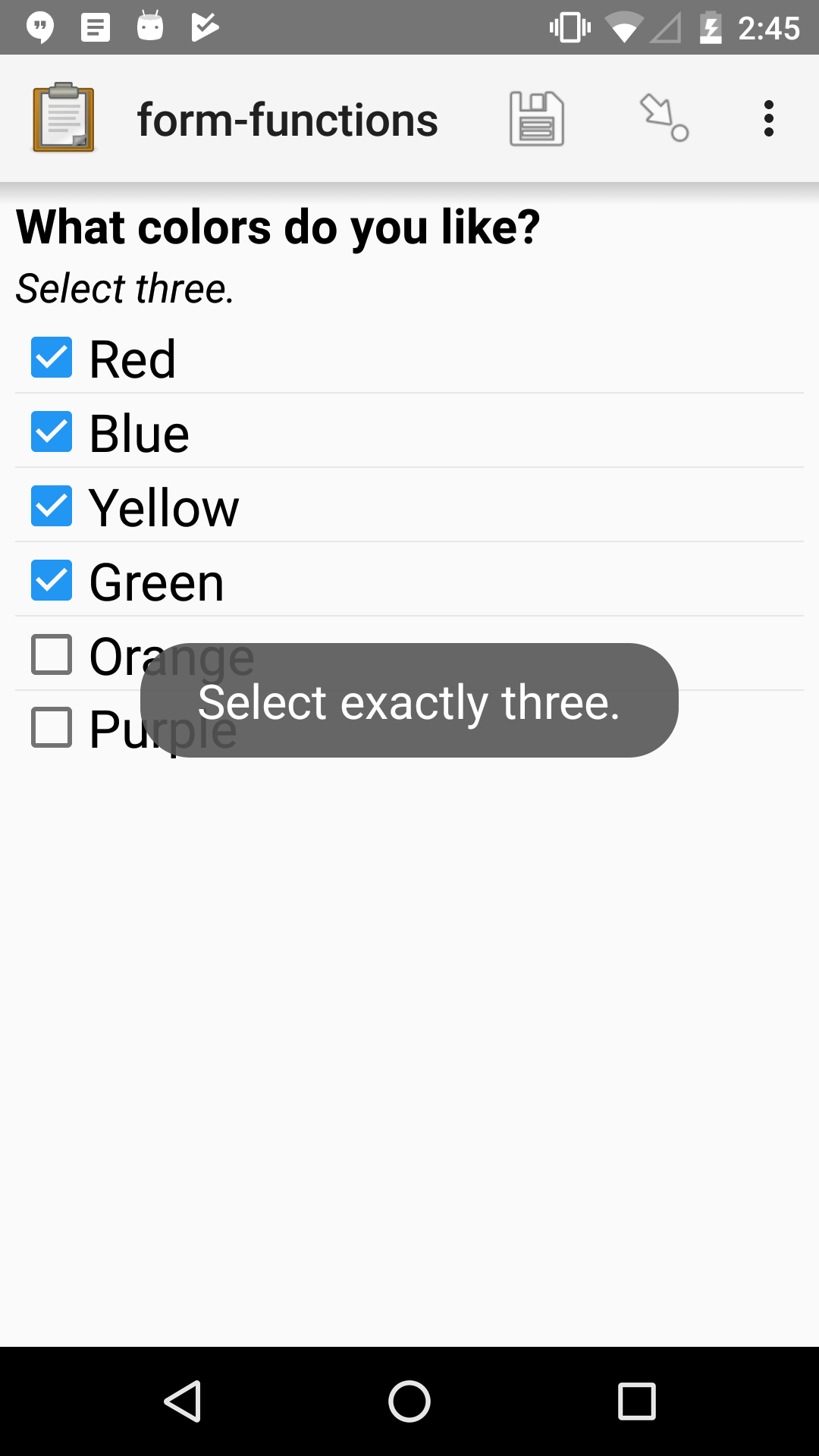XLSForm

survey#

type

name

label

hint

constraint

constraint_message

select_multiple colors

color_prefs

What colors do you like?

Select three.

count-selected(.)=3

Select exactly three.

choices#

list_name

name

label

colors

red

Red

colors

blue

Blue

colors

yellow

Yellow

colors

green

Green

colors

orange

Orange

colors

purple

Purple

jr:choice-name(choice_name, 'select_question')

Returns the label value, in the active language, associated with the `choice_name` in the list of choices for the `select_question`.

Note

You have to wrap the `select_question` reference in quotes.

```'\${question_name}'
```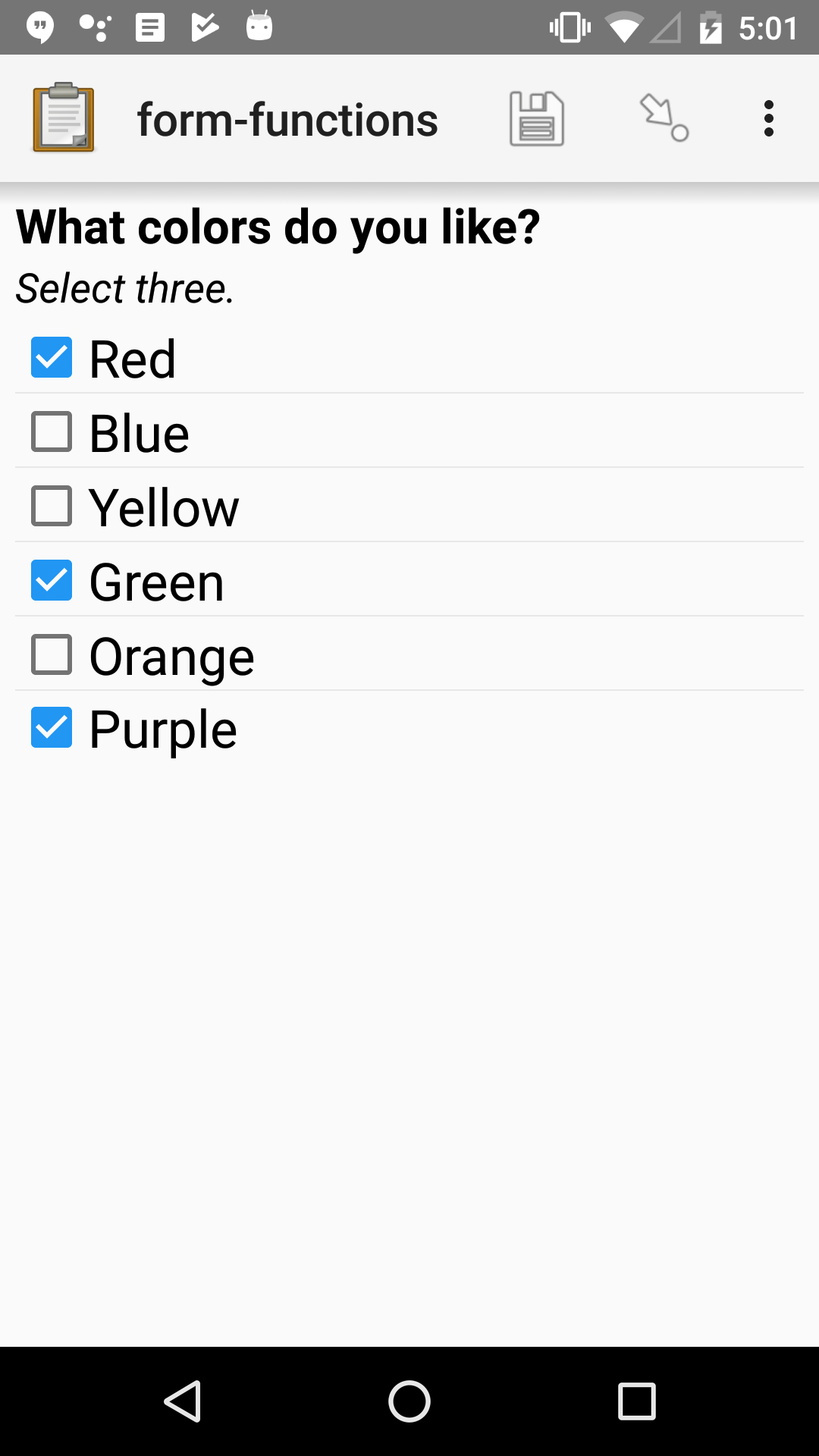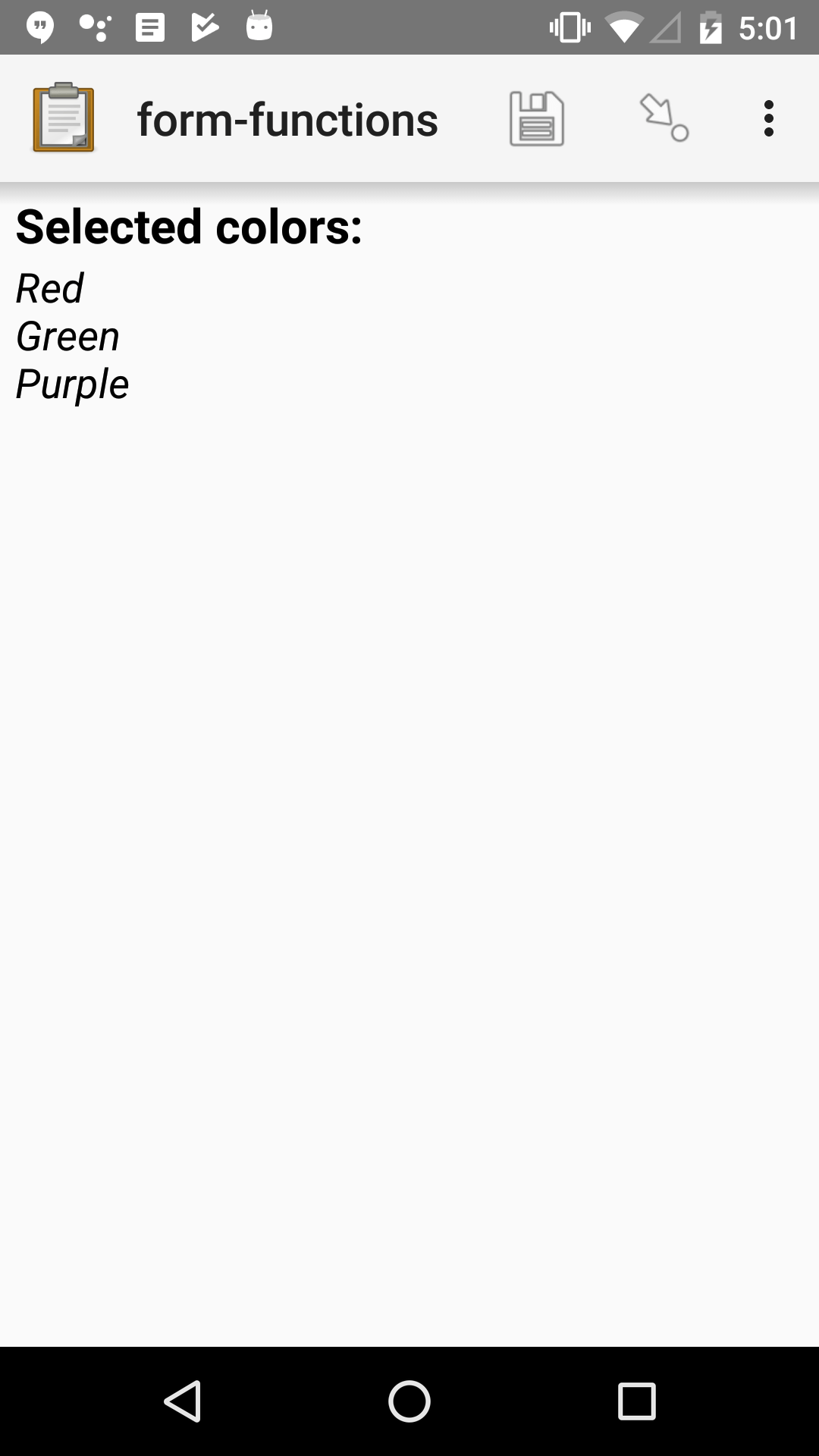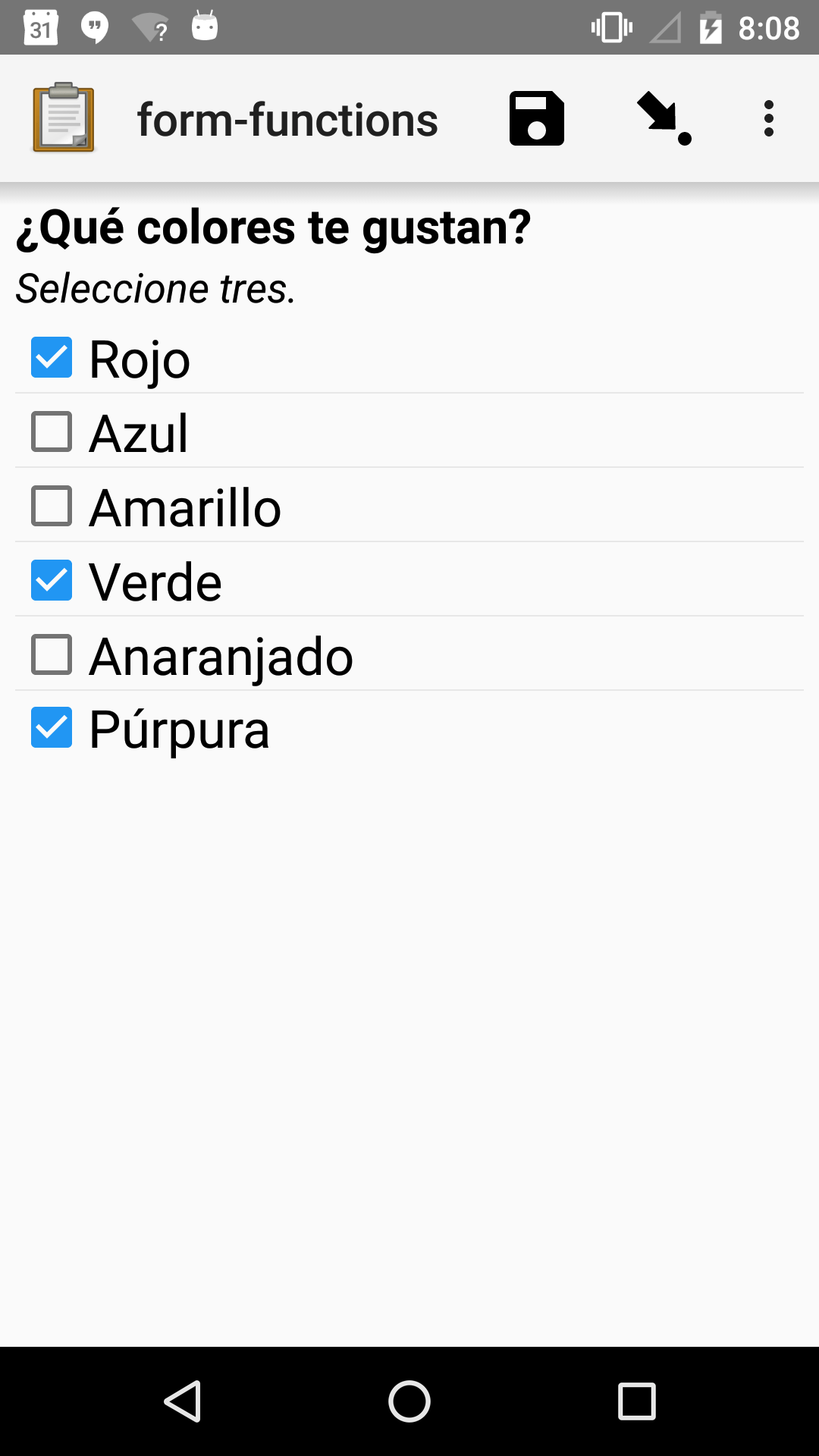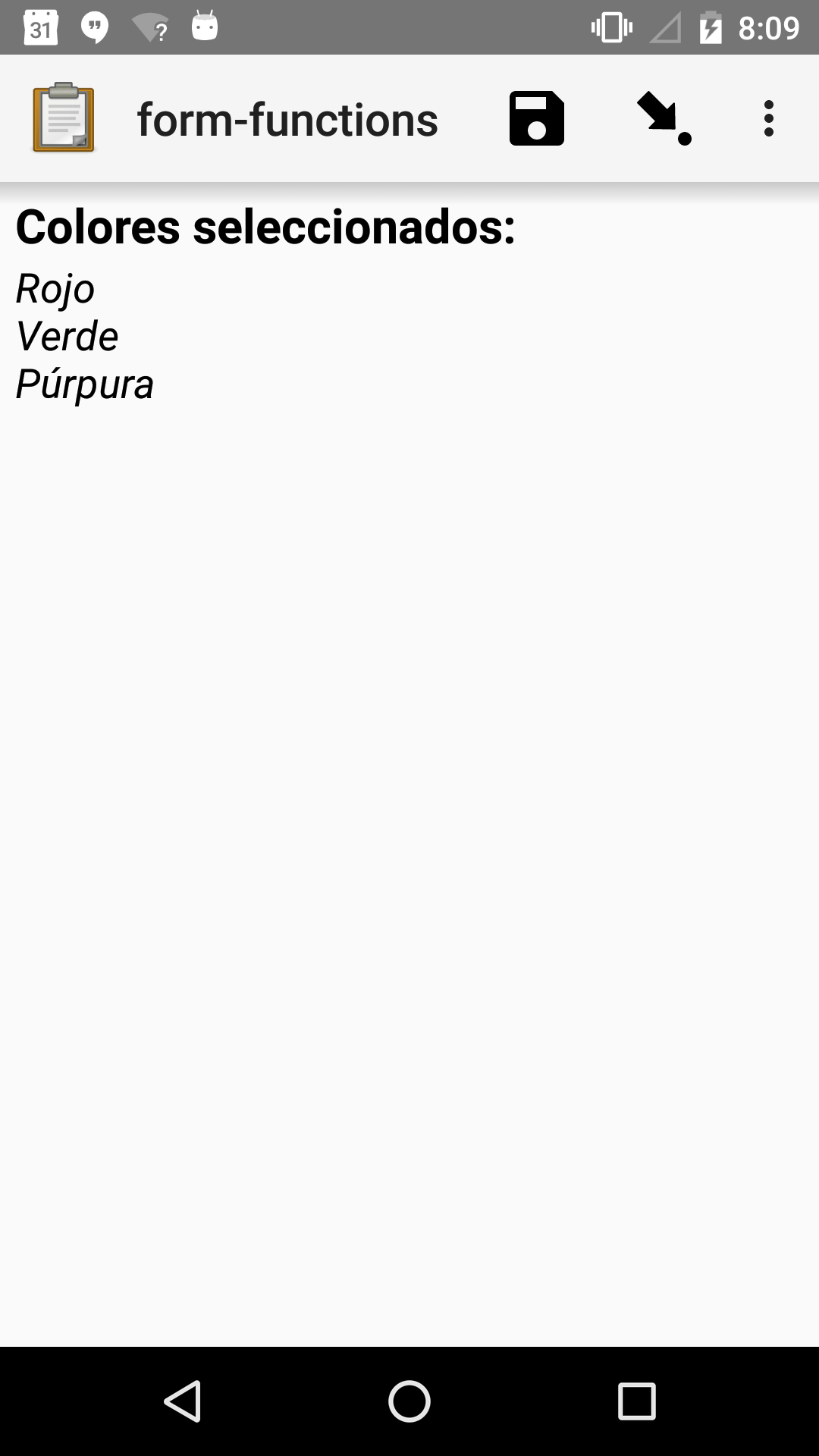XLSForm

survey#

type

name

label::English

label::Español

hint::English

hint:Español

calculation

select_multiple colors

color_prefs

What colors do you like?

¿Qué colores te gustan?

Select three.

Seleccione tres.

calculate

color_0

jr:choice-name( selected-at(\${color_prefs}, 0), '\${color_prefs}')

calculate

color_1

jr:choice-name( selected-at(\${color_prefs}, 1), '\${color_prefs}')

calculate

color_2

jr:choice-name( selected-at(\${color_prefs}, 2), '\${color_prefs}')

note

color_note

Selected colors:

\${color_0} <br> \${color_1} <br> \${color_2}

\${color_0} <br> \${color_1} <br> \${color_2}

choices#

list_name

name

label::English

label::Español

colors

red

Red

Rojo

colors

blue

Blue

Azul

colors

yellow

Yellow

Amarillo

colors

green

Green

Verde

colors

orange

Orange

colors

purple

Purple

Púrpura

#### Repeat groups#

nodeset#

A collection of XML nodes. In XLSForms, this is typically a collection of response values.

Outside a repeat group, referring to a question by name will return a nodeset containing all the responses to that question.

Nodesets can also be created by joining two or more nodes with pipes: `/data/age | /data/name`.

indexed-repeat(name, group, i [, sub_grp, sub_i [, sub_sub_grp, sub_sub_i ]])

Returns the response value of question `name` from the repeat-group `group`, in iteration `i`.

Nested repeat groups can be accessed using the `sub` and `sub_sub` parameters.

XLSForm

type

name

label

repeat_count

calculation

note

person_list_note

begin_repeat

person

Member of household

text

name

Name

end_repeat

begin_repeat

person_details

Details

count(\${person})

calculate

current_name

indexed-repeat(\${name}, \${person}, position(..))

date

member_bday

Birthday of \${current_name}

end_repeat

count(nodeset)#

Returns the number of items in `nodeset`. This can be used to count the number of repetitions in a repeat group.

XLSForm

type

name

label

repeat_count

calculation

note

person_list_note

begin_repeat

person

Member of household

text

name

Name

end_repeat

begin_repeat

person_details

Details

count(\${person})

calculate

current_name

indexed-repeat(\${name}, \${person}, position(..))

date

member_bday

Birthday of \${current_name}

end_repeat

count-non-empty(nodeset)

Returns the number of non-empty members of `nodeset`.

sum(nodeset)#

Returns the sum of the members of `nodeset`.

Can be used to tally responses to a repeated select question.

XLSForm

survey#

type

name

label

calculation

begin_repeat

guest_details

Guest details

text

guest_name

Guest name

select_one meal_options

meal_preference

Meal preference

calculate

chkn

if(\${meal_preference} = 'chicken', 1, 0 )

calculate

fsh

if(\${meal_preference} = 'fish', 1, 0 )

calculate

veg

if(\${meal_preference} = 'vegetarian', 1, 0 )

end_repeat

calculate

chkn_count

sum(\${chkn})

calculate

fsh_count

sum(\${fsh})

calculate

veg_count

sum(\${veg})

choices#

list_name

name

label

meal_options

chicken

Chicken

meal_options

fish

Fish

meal_options

vegetarian

Vegetarian

max(nodeset)#

Returns the largest member of `nodeset`.

XLSForm

survey#

type

name

label

calculation

begin_repeat

child_questions

text

child_name

Child's name

integer

child_age

Child's age

end_repeat

calculate

age_of_oldest_child

max(\${child_age})

min(nodeset)#

Returns the smallest member of `nodeset`.

XLSForm

survey#

type

name

label

calculation

begin_repeat

child_questions

text

child_name

Child's name

integer

child_age

Child's age

end_repeat

calculate

age_of_youngest_child

min(\${child_age})

Warning

The `min()` and `max()` functions only work sets of numbers. Empty values (that is, variables referencing unanswered questions) are actually empty strings, and will not be automatically converted to zero (0).

### Strings#

#### Searching and matching strings#

regex(string, expression)#

Returns `True` if `string` is an exact and complete match for `expression`.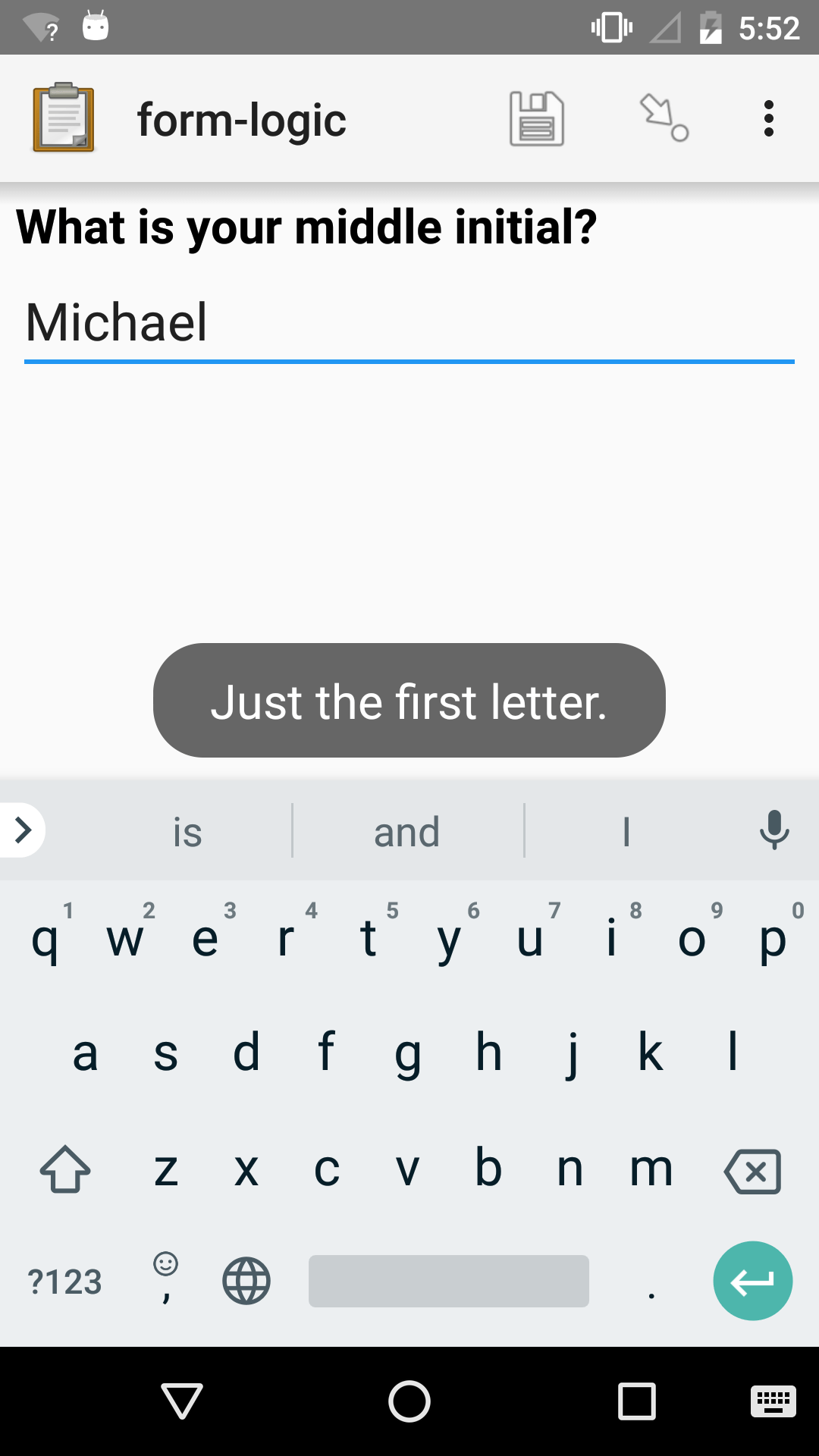XLSForm

survey#

type

name

label

constraint

constraint_message

text

middle_initial

regex(., '\p{L}')

Just the first letter.

contains(string, substring)#

Returns `True` if the `string` contains the `substring`.

starts-with(string, substring)

Returns `True` if `string` begins with `substring`.

ends-with(string, substring)

Returns `True` if the `string` ends with `substring`.

substr(string, start[, end])#

Returns the substring of `string` beginning at the index `start` and extending to (but not including) index `end` (or to the termination of `string`, if `end` is not provided). Members of `string` are zero-indexed.

substring-before(string, target)

Returns the substring of `string` before the first occurrence of the `target` substring. If the `target` is not found, or `string` begins with the `target` substring, then this will return an empty string.

substring-after(string, target)

Returns the substring of `string` after the first occurrence of the `target` substring. If the `target` is not found this will return an empty string.

translate(string, fromchars, tochars)#

Returns a copy of `string`, where every occurrence of a character in `fromchars` is replaced by the corresponding character in `tochars`. If `fromchars` is longer than `tochars` then every occurrence of a character in `fromchars` that does not have a corresponding character in `tochars` will be removed.

string-length(string)

Returns the number of characters in `string`. If no value is passed in, returns the number of characters in the value of the question that this function call is tied to which can be useful in a `constraint` expression.

normalize-space(string)

Returns a string with normalized whitespace by stripping leading and trailing whitespace of `string` and replacing sequences of whitespace characters with a single space. If no value is passed in, normalizes whitespace of the value of the question that this function call is tied to.

#### Combining strings#

concat(arg [, arg [, arg [, arg [...]]]])#

Concatenates one or more arguments into a single string. If any `arg` is a nodeset, the values within the set are concatenated into a string.

join(separator, nodeset)#

Joins the members of `nodeset`, using the string `separator`.

#### Converting to and from strings#

boolean-from-string(string)

Returns `True` if `string` is "true" or "1". Otherwise, `False`.

string(arg)#

Converts `arg` to a string.

### Math#

Warning

Math functions (except `number()`) only work with number values.

You can use `number()` to convert a string of digits to a number, but it is usually better to get a number value directly.

Empty values (that is, variables referencing unanswered questions) are actually empty strings, and will not be automatically converted to zero (0).

#### Number handling#

round(number, places)#

Rounds a decimal `number` to some number of decimal `places`.

int(number)#

Truncates the fractional portion of a decimal `number` to return an integer.

number(arg)#

Converts `arg` to number value.

If `arg` is a string of digits, returns the number value.

If `arg` is `True`, returns 1. If `arg` is `False`, returns 0.

If `arg` cannot be converted, returns `NaN` (not a number).

digest(data, algorithm, encoding method (optional))#

Computes and returns the hash value of the data `string` using the indicated hash algorithm `string`, and encoding this hash value using the optional encoding `string`.

Options for the algorithm are `MD5`, `SHA-1`, `SHA-256`, `SHA-384`, `SHA-512`.

If the third parameter is not specified, the encoding is `base64`. Valid options for the encoding are `base64` and `hex`.

This function can be useful if, for example, someone wants to build a unique identifier from sensitive data like a national ID number without compromising that data.

#### Calculation#

pow(number, power)#

Raises a `number` to a `power`.

log(number)#

Returns the natural log of `number`.

log10(number)#

Returns the base-10 log of `number`.

abs(number)#

Returns the absolute value of `number`.

sin(number)#

Returns the sine of `number`.

cos(number)#

Returns the cosine of `number`.

tan(number)#

Returns the tangent of `number`.

asin(number)#

Returns the arc sine of `number`.

acos(number)#

Returns the arc cosine of `number`.

atan(number)#

Returns the arctan of `number`.

atan2(y, x)#

Returns the multi-valued inverse tangent of `y`, `x`.

sqrt(number)#

Returns the square root of `number`.

exp(x)#

Returns `e^x`.

exp10(x)#

Returns `10^x`.

pi()#

Returns an approximation of the mathematical constant π.

### Date and time#

today()#

Returns the current date without a time component.

now()#

Returns the current datetime in ISO 8601 format, including the timezone.

Warning

#### Converting dates and time#

decimal-date-time(dateTime)

Converts `dateTime` value to the number of days since January 1, 1970 (the Unix Epoch).

This is the inverse of `date()`.

date(days)#

Converts an integer representing a number of `days` from January 1, 1970 (the Unix Epoch) to a standard date value.

This is the inverse of `decimal-date-time()`.

decimal-time(time)

Converts `time` to a number representing a fractional day. For example, noon is 0.5 and 6:00 PM is 0.75.

#### Formatting dates and times for display#

format-date(date, format)

Returns `date` as a string formatted as defined by `format`.

The following identifiers are used in the `format` string:

 %Y 4-digit year %y 2-digit year %m 0-padded month %n numeric month %b short text month (Jan, Feb, Mar…) %d 0-padded day of month %e day of month %a short text day (Sun, Mon, Tue…).

Note

Month and day abbreviations are language and locale specific. If form locale can be determined, that locale will be used. Otherwise, the device locale will be used.

format-date-time(dateTime, format)

Returns `dateTime` as a string formatted as defined by `format`.

The identifiers list in `format-date()` are available, plus the following:

### Geography#

area(nodeset | geoshape)#

Returns the area, in square meters, of either a `nodeset` of geopoints or a `geoshape` value.

It takes into account the circumference of the Earth around the Equator but does not take altitude into account.

distance(nodeset | geoshape | geotrace)#

Returns the distance, in meters, of either:

• a `nodeset` of geopoints

survey#

type

name

label

calculation

begin_repeat

points

geopoint

point

Record a Geopoint

end_repeat

calculate

calculated_distance

distance(\${point})

note

display_distance

Calculated distance: \${calculated_distance}

• the perimeter of a `geoshape`

survey#

type

name

label

calculation

geoshape

shape

Record a Geoshape

calculate

calculated_distance

distance(\${shape})

note

display_distance

Calculated distance: \${calculated_distance}

• the length of a `geotrace` value

survey#

type

name

label

calculation

geotrace

trace

Record a Geotrace

calculate

calculated_distance

distance(\${trace})

note

display_distance

Calculated distance: \${calculated_distance}

• concatenated geopoints

survey#

type

name

label

calculation

geopoint

point1

Record a Geopoint

geopoint

point2

Record a Geopoint

calculate

concatenated_points

concat(\${point1},' ; ', \${point2})

calculate

calculated_distance

distance(\${concatenated_points})

note

display_distance

Calculated distance: \${calculated_distance}

It takes into account the circumference of the Earth around the Equator and does not take altitude into account.

### Utility#

random()#

Returns a random number between 0.0 (inclusive) and 1.0 (exclusive).

Warning

randomize(nodeset[, seed])#

Returns a shuffled `nodeset`.

A shuffle with a numeric `seed` is deterministic and reproducible.

The primary use for this function is to randomize the order of choices for a select question. The documentation on select widgets describes how this is done in XLSForm.

`randomize()` can only be used in a context where a `nodeset` is accepted. Note that questions of type calculate cannot reference a `nodeset`.

uuid([length])#

Without argument, returns a random RFC 4122 version 4 compliant UUID.

With an argument it returns a random GUID of specified `length`.

boolean(arg)#

Returns `True` if `arg` is:

• a number other than zero

• a non-empty string

• a non-empty collection

• a comparison or expressions that evaluates to `True`.

Returns `False` if `arg` is:

• the number 0

• an empty string

• an empty collection

• a comparison or expression that evaluates to `False`.

not(arg)#

Returns the opposite of `boolean(arg)`.

coalesce(arg1, arg2)#

Returns first non-empty value of the two `arg` s. Returns an empty string if both are empty or non-existent.

checklist(min, max, response[, response[, response[, ...]]])#

Returns `True` if the number of `response` s that are exactly the string "yes" is between `min` and `max`, inclusive.

Set `min` or `max` to `-1` to make the argument not applicable.

weighted-checklist(min, max, reponse, weight[, response, weight[, response, weight[, response, weight[, ... ]]])

Returns `True` if the sum of the `weight` s of each `response` that is exactly the string "yes" is between `min` and `max`, inclusive.

Set `min` or `max` to `-1` to make the argument not

true()#

Evaluates to `True`.

false()#

Evaluates to `False`.